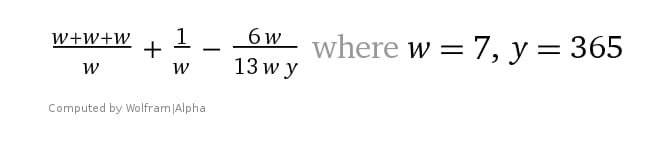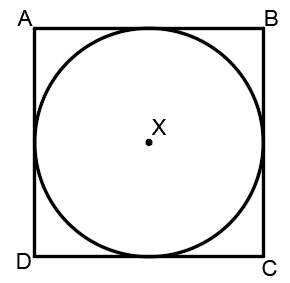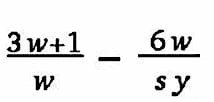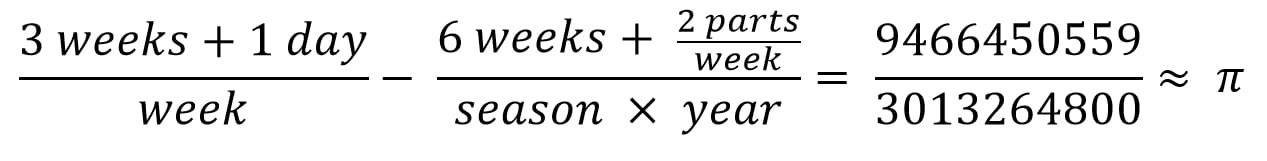# Pi as demonstrated in the life of Christ

In Part 3a of Pi-of-Time, it was demonstrated that the festivals of the Bible are derived from the formula for Pi, more precisely, from the formula for the “Pi-of-Time”.Pi-of-Time formula, accurate to Pi to the 18th decimal and produces all elements of time-keeping in one simple formula

The base unit of the formula is in weeks (“w”). But we also said that the “w” can represent weeks of days or weeks of years, just as in the Bible, (Daniel 9:24-27, etc.). In other words, a day can symbolize a year of time, (Ezekiel 4).

So far we looked at the regular week composed of seven days. We paid special attention to these seven days in relation to the seven days of Creation and to the seven days of Passover, and especially Tabernacles. We also looked at Pentecost.

But something interesting happens when we convert units of days to years. Let’s look at the first term in the second part of the formula. Below we rewrite it a little differently, using seven weeks (“w”).Notice that “13 w y” of the denominator equals 33215. The numerator (at top) is what tells us the circumference of a circle in ratio to one side of a square. One must multiply the numerator by four to obtain the perimeter of the square that circumscribes the circle. This yields a fraction of 104348/(33215 x 4) “days”.circumscribed square of a circle is a square surrounding a circle such that the circumference of the circle touches the midpoints of the four sides of the square. The diameter of the circle is equal to the side length of the square. (Wiki)

The fraction of 104348/33215 as a decimal is 3.141592654 whereas Pi is 3.1415926536. Extremely accurate for such a small equation.

We already explained the Enoch Calendar and how it works. Pi is rooted in the Enoch Calendar.  It is composed of exactly 52 weeks, and each season is exactly 13 weeks. Thus there are exactly 364 days in an Enochian year.

The Bible tells us that Enoch lived “365 years”. Therefore, both “364” (as per the Book of Enoch) and “365” (as per the Bible) are fundamental to the Enoch Calendar, (Gen. 5: 21-24). The fact that Enoch’s grandson was half-364 years of age when Noah was born demonstrates that in the Bible, both 364 and 365 are acknowledged as solar years, (Gen. 5:28-31).

Moreover, Enoch’s grandson lived “777 years” in total, again demonstrating the importance of “seven”, so fundamental to Enoch’s calendar. (See “Enoch Calendar” for more examples of a 364-year in the Bible.)Having reiterated the fundamentals of Enoch’s Calendar, let us examine the Pi formula again. We said that the ratio (fraction) for Pi at this early stage in the formula is 104348/(33215 x 4). We said that 33215 x 4 is the perimeter of the square and 104348 is the circumference.

33215 x 4” equals 132860 “days”. It happens that 132860 days is exactly 364 x 365 days! (365 x 365 = 132860). Thus, the formula itself implies that day is as a year and a year is as a day! (Thus, because Enoch lived 365 years, thus all the days of Enoch’s life are 33215 days!)

And this is also the most accurate rationalization of Pi possible with so few digits in the fraction.

One is left either with an incredible coincidence wherein “364 years” (of 365 days) happens to be inherent in Pi, or else God determined it so.

Remember, each side of the square, therefore, depicts one season of the year, either as 91 or 91.5 days! And the seasons are broken down into weeks, and so forth.

What are the odds!

We can rewrite the same formula to reference seasons:“s y” stands for ‘a season times a year’, and equals the number of days that Enoch lived, 33215 days, and “coincidentally” is Pi to the 8th decimal!

To convert days to years (as implied in the formula itself) we multiply the fraction 104348/33215 by 364, which equals 1144 days less 13 hours. (More precisely, 1143 days, 11 hours, 168 seconds.)

But back up and notice that the first term by itself, “(3w+1)/w” (i.e., 22/7) multiplied by 364 days equals exactly 1144 days. (22/7 x 364 = 1144.) Thus, “6w” in units of years of 364 days loses about half-a-day every 1144 days.

From the anniversary of the first day of Creation, when God’s Holy Spirit “hovered over the face of the waters”, until Pentecost when God “poured out the Holy Spirit” are the 1144 days of Pi. Pi, in this case, represents the square and compass, so to speak, of God’s Creation.

Who can fathom the Spirit of the Lord,

or instruct the Lord as his counselor?…

Do you not know?

Have you not heard?

Has it not been told you from the beginning?

Have you not understood since the earth was founded?

He sits enthroned above the circle of the earth,

and its people are like grasshoppers.

He stretches out the heavens like a canopy,

and spreads them out like a tent to live in. (Isaiah 40:13, 21-22)

The circle is at the heart of all these fractal images.

God’s fingerprints are in the Pi formula for a circle — God’s creation in one week, (Gen 1:14-16; 2:2).Astonishingly, this formula is accurate to 12 decimals of Pi. It encodes every aspect of our calendar year, including seconds, “parts“, days, weeks, months, seasons, years, Sabbath years, jubilees, even the vast “Years” of Precession of 25920 years, etc. It also includes all the Jewish festivals of the Bible. This could be the greatest evidence ever given to man that God exists and created all things. If you know someone with even a basic understanding of math or astronomy, please email the formula to them along with this brief explanation!

In the above formula, “2 parts/7” refers to the smallest part of a day according to Jewish and Babylonian reckoning, and in this case, equals the biblical “blink of an eye”.

The numerator (circumference) equals “≈ 1000 Years of Precession as if one day, and a day as if 1000 Years”, 2Pet 3:8.

The denominator produces the perimeter of the square. It equals 1274 Years of Precession of 365 days per year. (“1274” is Enoch’s equivalent of 1260, Rev. 12:6.)